MathBox Examples

This webpage contains a collection of interactive mathematical visualizations built using MathBox: a library for rendering presentation-quality math diagrams in a browser using WebGL, built on top of Three.js and ShaderGraph. Most examples also utilize dat.gui for the user interface.
This collection is hosted at GitHub.
Last updated: 18 June 2016.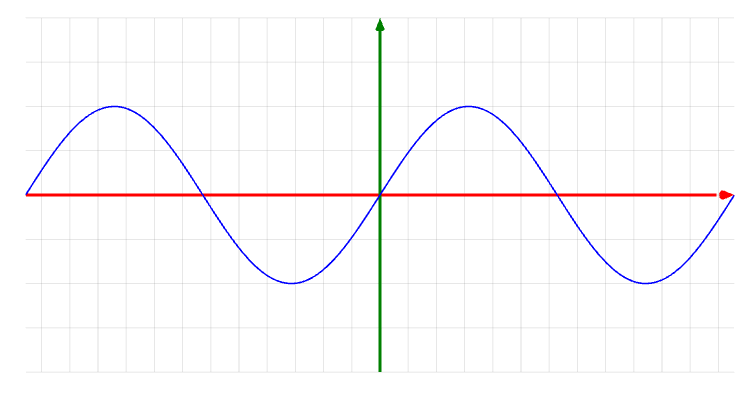MathBox Introduction

Illustrates how to create a graph of a 2D function y = f(x) in MathBox.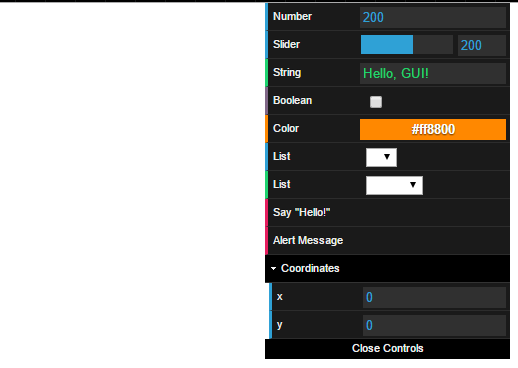dat.gui Demo

Illustrates how to set up the different data types using dat.gui: numbers, number sliders, text, booleans, colors, lists, functions, and folders.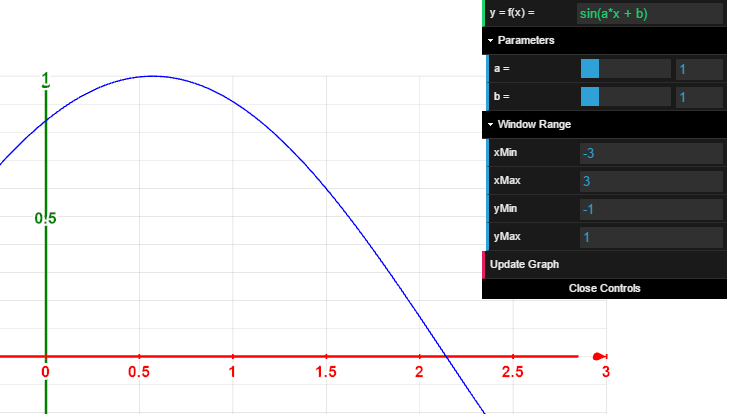Integrating MathBox with dat.gui

Graph of a 2D function y = f(x). New features:

• integration with dat.gui
• function may be entered via text box; formula parsed via Silent Matt's JavaScript Expression Evaluator
• adjustable parameters a and b can be used in the function definition
• axes contain tick marks and corresponding labels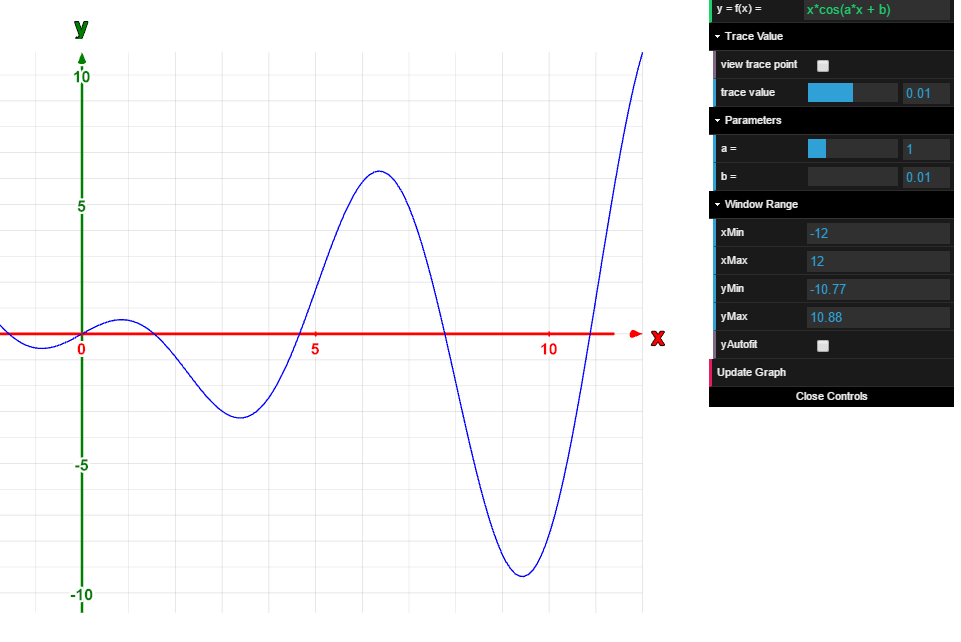2D Function Graph

Graph of a 2D function y = f(x). New features:

• a trace point whose location is controlled by a slider, visibility is controlled by a textbox, and a label below the trace point displays its coordinates to two decimal places
• axes are labelled, and y-bounds can be set to automatically fit themselves according to the minimum and maximum values of the function with the x-bounds.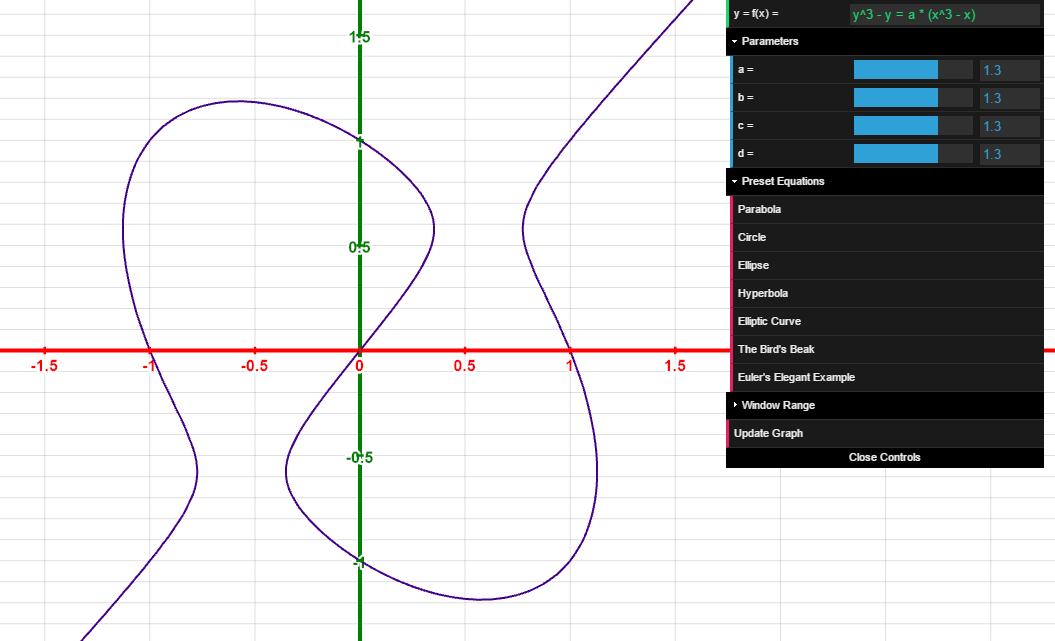2D Implicit Curve Graph

Graph of a 2D curve defined implicitly by f(x,y) = g(x,y). Uses the marching squares algorithm to approximate the curve. Includes a variety of preset equations.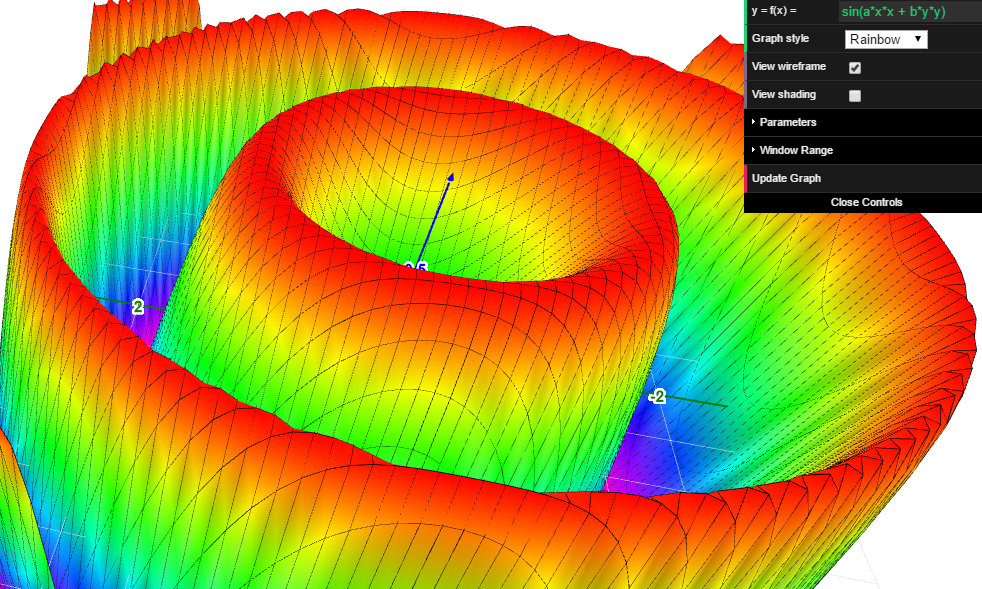3D Function Graph

Graph of a 3D function z = f(x, y). Includes adjustable parameters, various color schemes, and optional wireframe and shading.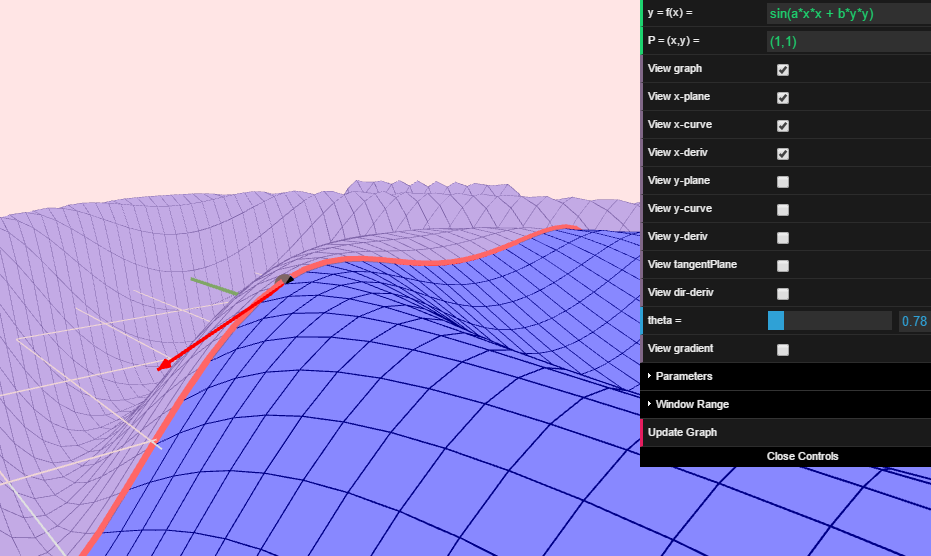3D Derivatives

Graph of a 3D function z = f(x,y). Coordinates of a point entered as text. Includes optional graphs of x- and y- partial derivatives, tangent plane, directional derivatives, and the gradient at the given point.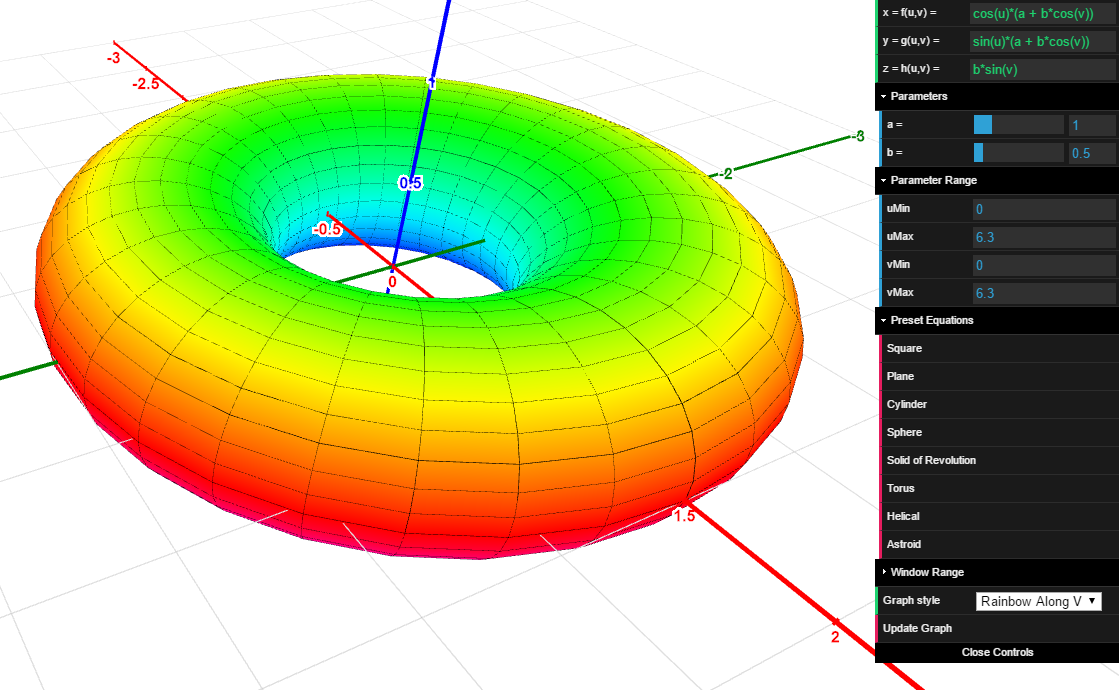Surfaces in 3D

Graph of a 2D surface (x,y,z) = (f(u,v), g(u,v), h(u,v)) in 3D.Curves in 3D

Graph of a curve (x,y,z) = (f(t), g(t), h(t)) in 3D. Plots a variable size/color tube around the curve for easier visibility.